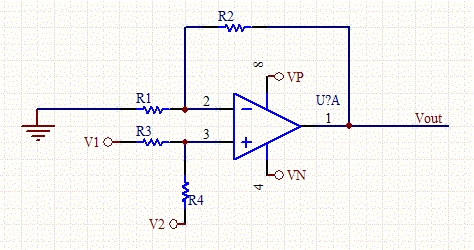# Non-Inverting Op-Amp Resistor Calculator

This calculator determines the bias and feedback resistors for a non-inverting op-amp, given the gain and desired output bias point. There are many free parameters to the design so enter the value of R1, which will scale the other resistors. Use V1 as the input for the inverting Op-Amp, and V2 as a voltage offset if needed. Set V2 to zero if no offset is required.

To use the calculator you first need to determine the desired gain of the ac signal, and the quiescent output voltage (the value when the AC input signal is zero).

R4 injects a non-zero V2 voltage component into the circuit and correspondingly offset Vout. Another way of looking at it is, if V2 is zero and the DC component of V1 is zero then Vout will also be zero. The amplified output signal will ride on the quiescent output voltage.Non-Inverting Op-Amp Circuit Schematic

 Input Parameters (Non-Inverting Op-amp) Vout (V) Gain R1 (KOhms) V1(Input lead) (V) V2 (non-zero only if offset is requred) (V) Vp (V) Vn (V) Outputs R2 (KOhms) R3 (KOhms) R4 (KOhms)

[Plant Database], [Soil Moisture Sensor] [Water Level Sensor] [Soil Moisture Meter]